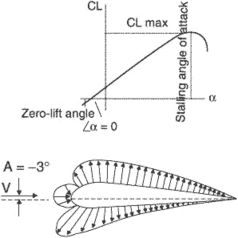zero-lift angle

zero-lift angle

[′zir·ō ¦lift ′aŋ·gəl]
(aerospace engineering)
The angle of attack of an airfoil when its lift is zero.

zero-lift anglePressure distribution on an airfoil at the angle for zero lift (−3°).
The angle of attack at which an airfoil does not produce any lift. Its value is generally less than zero unless the airfoil is symmetrical.
Site: Follow: Share:
Open / Close